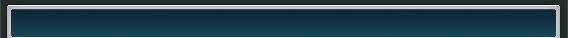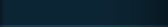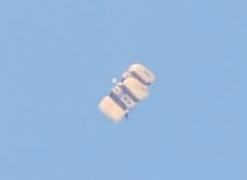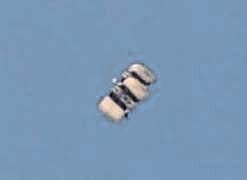Home%    Dynamic Range Compaction
%   This routine reads a color image, imposes a non-linear dynamic range
%   stretch per routine compaction, and writes out a modified image

%   Read in image and separate out the intensity channel

hsv_image=rgb2hsv(color_image_input);
image_input=uint8(255.*hsv_image(:,:,3));

%   Call the non-linear compaction routine

[new_image,remap]=compaction(image_input);

%   Re-introduce the new intensity channel and create the output image

hsv_output_image=hsv_image;
hsv_output_image(:,:,3)=double(new_image)./255;
color_output_image=hsv2rgb(hsv_output_image);

%   Display and write out the new image

figure
imagesc(color_output_image);

imwrite(color_output_image,'test_output.jpg','jpg');

function [new_image, remap] = compaction (image_input)

%    Image compaction algorithm

%        Routine eliminates all zero content bins in the image histogram and
%        moves values in bins separated by zero bins to immediately adjacent bins.
%        This creates a non-linear but reversible contrast stretch that maintains
%       visual qualitative brightness of pixels without causing contouring or other
%       artifacts.

%    Calculate histogram

[m n]=size(image_input);

%   Set to 256 for 8 bit image

histogram=imhist(image_input,256);
length_of_histogram=length(histogram);

%   Determine how to remap pixel values from old to new image

remap(1:length_of_histogram)=1;
remap_index=1;
for i=1:length_of_histogram,
if (histogram(i)>0),
remap_index=remap_index+1;
remap(i)=remap_index;
end;
end;

%    Remap old image values to new image values

for i=1:m,
for j=1:n,
temp_new_image(i,j)=remap(double(image_input(i,j))+1)-1;
end;
end;

%    Stretch image to 8 bit dynamic range

new_image=uint8(temp_new_image.*255./max(max(temp_new_image)));
%clear temp_new_image;

%    Display remapped image

figure
imagesc (new_image)
colormap(gray)Before
After
 Serious Science LLC            Where science fiction becomes fact.